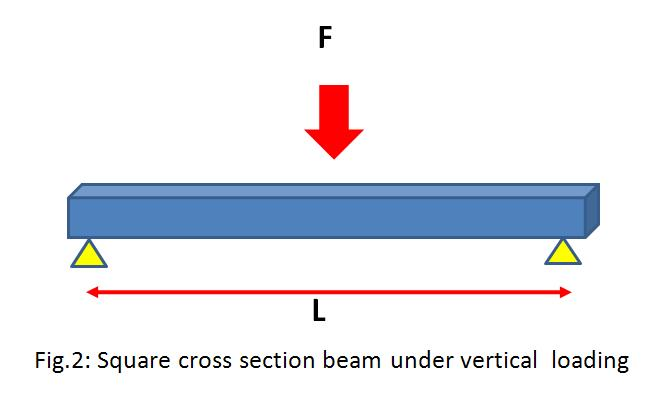# How to Select Materials for Your Mechanical Design

Here, we will discuss the material selection process for the mechanical design of the following three basic types of structural members:

b)      Square cross section beam under vertical  loading

c)       Rectangular cross section panel under vertical loading

Of course, the real life design problems are very much complicated, but I think these basic examples are the building blocks for the complicated problems.

a) Material Selection Guide for Circular Cross Section tie-road Design• F – Axial force
• L – Length of the tie-rod
• A – Cross section area of the tie rod
• δ – axial deformation of the tie road
• d- density
• s- stress
• m – mass of the tie rod
• E – Modulus of elasticity

We have the following two basic equations:

δ =LF/AE……………………………..eqn.1

m=AL/d…………………………….eqn.2

From the above two equations, we can write:

m= (L2F/ δ) (d/E)…………………..eqn.3

As a designer, our aim is to minimize the mass of the tie rod and to achieve that we have to minimize the quantity (d/E).

So, for the design of tie rod under axial loading the material with minimum (d/E) has to be chosen.

b) Material Selection Guide for Square Cross Section Beam Design• F – Axial force
• L – Length of the beam
• b – Length of one side of the cross section area of the beam
• δ– Deflection  of the beam
• d- Density
• s- Stress
• m – Mass of the beam
• B – Stiffness of the beam
• I  – Area moment of inertia for the beam
• K – Constant
• E – Modulus of elasticity

We have the following two basic equations:

B = F/ δ = KEI/L3=KEb4/12L3……………………………..eqn.4

m= b2Ld……………………………………..…………………..eqn.5

Combining the above two equations we get

m = (12BL5/K)1/2(d/E1/2)………………………………eqn.6

So, in case of designing the beam under vertical loading for achieving the minimum mass, we have to minimize the quantity (d/E1/2) .

c) Material Selection Guide for Rectangular cross section• F – Axial force
• L – Length of the beam
• b – Width of the panel
• t – Thickness of the panel
• δ– Deflection  of the beam
• d- Density
• s- Stress
• m – Mass of the beam
• B – Stiffness of the beam
• I  – Area moment of inertia for the beam
• K – Constant
• E – Modulus of elasticity

We have the following two basic equations:

B = F/ δ = KEI/L3=KEbt3/12L3……………………………..eqn.7

m= btLd……………………………………..…………………..eqn.8

Combining the above two equations we get

m = (12Bb2/K)1/3(d/E1/3)L2………………………………eqn.9

So, in case of designing the panel under vertical loading for achieving the minimum mass, we have to minimize the quantity (d/E1/3).

Material selection for mechanical design in case of tie-rod emphasis on the quantity (d/E), in case of designing the square beam the quantity of importance should be (d/E1/2) and in case of rectangular panel the quantity of importance should be (d/E1/3). Once, as a mechanical designer you are able to find out the material selection parameter, you can use the material selection tool or software like CES for actually selecting the most suitable material for your application. I will discuss the different steps required for using CES in my next article.

### Shibashis Ghosh

Hi, I am Shibashis, a blogger by passion and engineer by profession. I have written most of the articles for mechGuru.com. For more than a decades i am closely associated with the engineering design/manufacturing simulation technologies.
Disclaimer: I work for Altair. mechGuru.com is my personal blog. Although i have tried to put my neutral opinion while writing about different competitor's technologies, still i would like you to read the articles by keeping my background in mind.

## 3 Replies to “How to Select Materials for Your Mechanical Design”

1.I. C. E Akagu says:

When are you going to do your next article ?

2.I. C. E Akagu says:

Next article ?

3.Dhpr says:

excellent article
….send next…soooooooooooooon

This site uses Akismet to reduce spam. Learn how your comment data is processed.